# A New Approach to ICSE Physics Part 2 Class 10 Solutions Machines

## A New Approach to ICSE Physics Part 2 Class 10 Solutions Machines

These Solutions are part of A New Approach to ICSE Physics Part 2 Class 10 Solutions. Here we have given A New Approach to ICSE Physics Part 2 Class 10 Solutions Machines

Exercise – 1

Question 1.
(a) What is a machine ?
(b) State three functions of machine.
(a) Machine : “Is a device which is used to transmit force to change the direction of force or to obtain speed.”
(b) Functions of machines :

1. To act as force multiplier
2. To shift the point of application to a convenient place.
3. To multiply speed.
4. To change the direction of the force applied for greater ease and comfort.

Question 2.

(a) Name six simple machines.
(b) Give one practical example of each machine named in (a)

(a) Simple machines are :

1. Lever
2. Pulley
3. Wedge
4. Inclined plane
5. Wheel and axle
6. Screw

(b) Example of :

1. Liver : is handle of common water pump helps in multiplying effort
2. Pulley : gears, used for changing the direction of the effort
3. Wedge : axe, A knife are the examples.
4. Inclined plane : Ramp or staircase or screw jack.
5. Wheel and axle : Free wheel or crank wheel of bicycle.
6. Screw : Screw bolt.

Question 3.
Define the following terms with reference to a machine

(c) effort
(d) ideal machine
(g) velocity ratio
(h) efficiency

(a) Total load : Load lifted by machine + resistance over come.
(b) Useful load : “The load, a machine in able to lift.”
(c) Effort : The effort applied by us on the machine.
(d) Ideal machine : A machine whose parts are weightless and frictionless such that what so ever is the energy spent on it same is the energy produced by it, is called a perfect machine.”
(e) Ideal mechanical advantage : “The ratio between total load moved (or resistance overcome) to the effort applied is called ideal mechanical advantage.”
(f) Actual mechanical advantage : “The ratio between useful load moved to the effort applied is called actual mechanical advantage”.
(g) Velocity ratio : [V.R.] : “Is the ratio of velocity of effort to velocity of load.”
i. e “Ratio of effort arm to load arm.”
(h) Efficiency : (η) “Efficiency of a machine is the ratio of useful work done by machine to the work put into the machine by the effort.”

Question 4.
Prove that efficiency of a machine is the ratio between mechanical advantage and velocity ratio.
Relation Between M.A., V.R And Efficiency :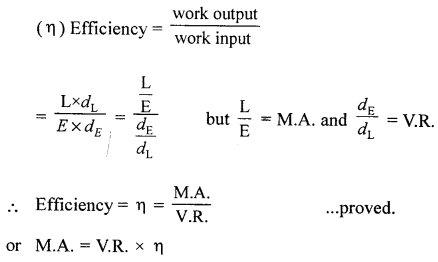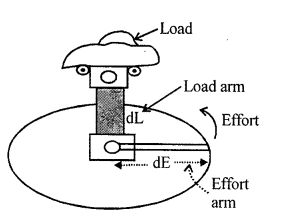Question 5.
Give two reasons, why machine cannot be 100% efficient.

1. Apart of input is always used in moving the parts of machine.
2. A part of input is wasted in overcoming friction between various parts of machine.

Question 6.
(a) Define lever. What are its kinds ?
(b) How will you determine the order of a lever ?
(a) Lever : “Is a straight or bent rigid rod which can turn about a fixed point called fulcrum”
(b) Out of effort (E), Load (L) and Fulcrum (F), the one which is in between the other two determines the order of lever i.e.
If F is in between L and E, then it is lever of 1 st order
If L is in between F and E. It is of second order lever.
If E is in between L and F, it is Illrd order lever.

Question 7.
To which order do the following levers belong and why ?

(a) A railway signal
(b) A man cutting bread with a knife
(c) A boy writing a piece of paper
(d) A nut cracker
(e) handle of a water pump
(f) see-saw
(g) forceps
(h) A man rowing a boat
(i) A lock and key
(j) Soda water opener
(k) a door
(l) motorcarfoot brake
(m) fishing rod
(n) lemon squeezer.

(a) A railway signals – Lever of 1st order as F is in between load and effort
(b) Cutting a bread with knife is third order lever as effort is in between load and fulcrum.(c) A boy writing a piece of paper is Illrd order lever as effort is in between load and fulcrum.
(d) A nut cracker is II nd order lever as load is in between effort and fulcrum.(e) Handle of water pump : 1st order lever as fulcrum is in between effort and load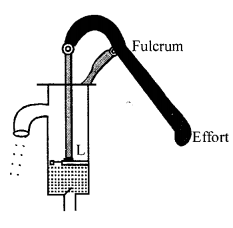(f) See-saw : Lever of 1st order as fulcrum is in between load and effort(g) Forceps : Illrd order lever as effort is in between fulcrum and load.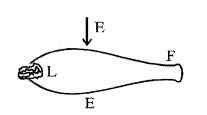(h) A man rowing a boat : End order of lever as load is in between effort and fulcrum(i) Bottle Opener : Ilnd order lever as load is in between fulcrum and effort.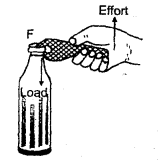(j) Soda – water opener : 2nd order lever as load is in between fulcrum and effort.
(k) A door : Ilnd order lever as load is in between effort and fulcrum.
(l) Motorcar foot brake : 3 rd order lever as effort is in between load and fulcrum.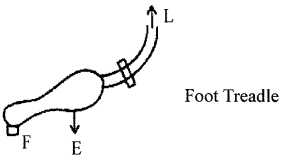(m) A fishing rod : 3 rd order lever as effort is in between fulcrum and load.
(n) Lemon squeezer : 2 nd class lever as load is in between fulcrum and effort.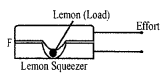Question 8.
Why does a lever of second order have mechanical advantage more than one ?
M.A. of second order of lever is more than one as effort arm is longer than load arm M.A = Effort arm / Load arm

Question 9.
Why does a lever of third order have a mechanical advantage less than 1 ?
M.A. of Illrd order lever is always less than 1 as effort arm is less than load arm M.A. = Effort arm / Load arm

Question 10.
In which situation does the lever of first order have mechanical advantage (a) more than 1 (b) less than 1 ?

(a) Mechanical advantage can be more than 1 when effort arm is larger than load arm or by moving the fulcrum towards the load.
(b) M.A. can be less than lwhen effort arm is smaller than load arm or by moving fulcrum towards effort.

Question 11.
Why are cutting edges of pliers smaller than the cutting edges of scissors ?
Cutting edges of pliers are smaller to make it force multiplier. Load arm is kept very small because the resistance (load) of the metal is very large and to over come this resistance the effort arm is made larger.

Multiple Choice Questions

Tick ( ✓ ) the most appropriate option.

1. Which of the statement is not true for a machine ?
(a) It can multiply force
(b) It can increase speed
(c) It can change the direction of applied force.
(d) Its output can be more than input.
(d) Its output can be more than input.

2. Which of the statement is not true for an actual machine?
(a) Its mechanical advantage is less than velocity ratio.
(b) Its efficiency is always less than 100%
(c) Its mechanical advantage is greater than velocity ratio.
(d) Output of machine is always less than input.
(c) Its mechanical advantage is greater than velocity ratio.

3. A lever which always has mechanical advantage less than 1 has :
(a) Load between effort and the fulcrum.
(b) Effort between the load and the fulcrum.
(c) Fulcrum between the load and effort.
(d) Effort and load act at same point.
(b) Effort between the load and the fulcrum.

4. The correct relationship between the effort (E) load (L) and mechanical advantage (M.A.) is :
(a) M.A. = L × E
(b) M.A. × E = L
(c) M.A. x L= E
(d) none of these
(b) M.A. × E = L

5. The correct relationship between the velocity ratio (V.R) the distance through which effort act (d) and the distance through which load moves (D)
(a) V.R. × D = d
(b) V.R. = D × d
(c) V.R. × d = D
(d) none of these
(a) V.R. × D = d

6. The correct relationship between the efficiency (η), mechanical advantage (M.A.) and velocity ratio (V.R.) of an actual machine is :
(a) η = M.A × V.R
(b) η = M.A. ÷ V.R.
(c) η × V.R. = M.A.
(d) both (b) and (c)
(c) η × V.R. = M.A.

7. A fire tong is a lever of :
(a) First order
(b) Second order
(c) Third order
(d) none of these
(c) Third order

8. A person standing vertically upon his toes is an example of lever of :
(a) First order
(b) Second order
(c) Third order
(d) none of these
(c) Third order

9. A lever which always have mechanical advantage more than 1 is :
(a) Lever of first order
(b) Lever of second order
(c) Lever of third order
(d) none of these
(b) Lever of second order

10. A lever which can have mechanical advantage either less than one or more than one depending upon the position of fulcrum is a lever of :
(a) First order
(b) Second order
(c) Third order
(d) none of these
(a) First order

Numerical Problems on Levers

Practice Problems 1

Question 1.
In operating a water pump, a resistance of 480 N is overcome by an effort of 72 N. If the distance of the fulcrum from the point where the resistance act is 0.2 m. Find the distance from the fulcrum where effort is applied.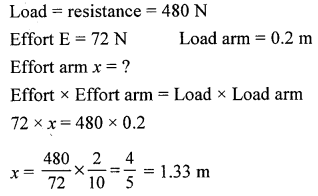Question 2.
A uniform plank of a see-saw is 5m long and supported at its centre. A boy weighing 40 kgf sits at a distance 1.5 m from one end of the see-saw where must a girl weighing 25 kgf sit on the other end of the see-saw so as to balance the boy ?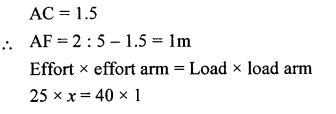Practice Problems 2

Question 1.
The handle of a nutcracker is 16 cm long and a nut is placed 2 cm from its hinge. If a force of 4 kgf is applied at the end of the handle to crack it, what weight, if simply, placed on the nut will crack it ?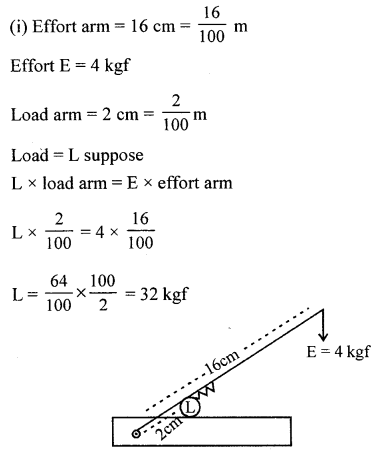Question 2.
An effort of 50 kgf is applied at the end of a lever of the second order, which supports a load of 750 kgf, such that the load is at a distance of 0.1 m from the hinge. Find the length of the lever. (Assume that the lever is weightless)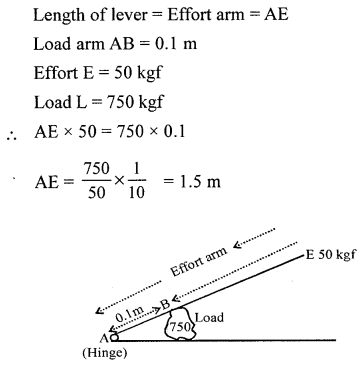Practice Problems 3

Question 1.
A machine displaces a load of 125 kgf through a distance 0. 30 m, when an effort of 12.5 kgf acts through a distance of 4.0 m. Calculate the

(a) velocity ratio
(c) % age efficiency of the machine.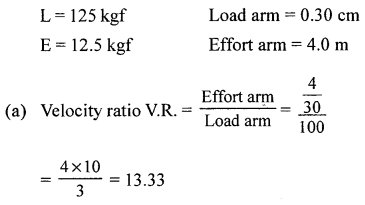Question 2.
Calculate the (a) velocity ratio (b) mechanical advantage (c) %age efficiency of a machine which overcomes a resistance of 800 N through a distance of 0.12 m, when an effort of 160 N acts through a distance of 0.72 m.Practice Problems 4

Question 1.
An effort of 500 N is applied through a distance of 0.50 m on a machine, whose efficiency is 90% such that resistance is overcome through a distance of 0.04 nuCalculate the (a) V.R. (b) M.A. (c) resistance overcome by the machine.Question 2.
A crow-bar of length 2.0 m is used as a machine, to lift a box of 100 kgf by placing a fulcrum at a distance of 0.1 m from the box Calculate the (a) V.R (b) effort required. What assumption has been made by you in solving the problem ?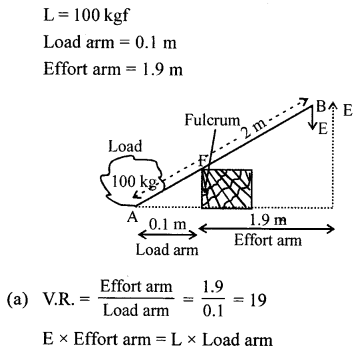Practice Problems 5

Question 1.
The handle of a water pump is 90 cm long from its piston rod. If the pivot of handle is at a distance of 15 cm from the piston rod, calculate :
(b) least effort required at its other end to overcome a resistance of 60 kgf.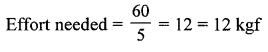Question 2.
A crowbar of length 2.5 m is pivoted at a point 25 cm from its tip. Calculate (i) mechanical advantage of crowbar (ii) the maximum load displaced by it by applying an effort of 100 kgf on its extreme end.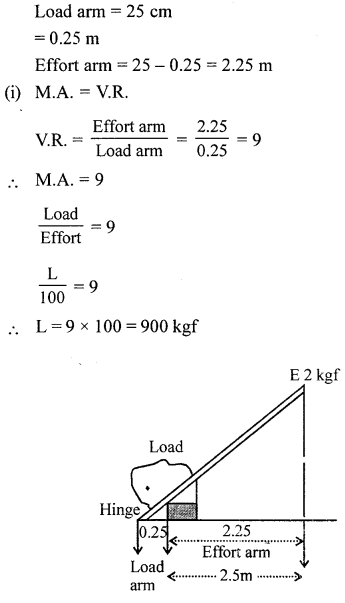Practice Problems 6

Question 1.
A walnut can be broken by applying a direct force of 50 kgf. If the walnut is placed in a nut crackers, the length of whose handle is 18 cm and nut is placed 2 cm from the pivot, calculate the minimum force required to crack the nut.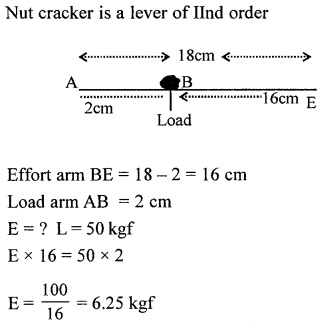Question 2.
A key is 5 cm long the turns the levers of a lock at a distance 1 cm. If the lock is opened by an effort of 10 N, calculate the resistance offered by the levers of the lock.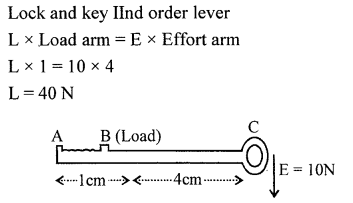Practice Problems 7

Question 1.
A door is 1 m wide. It can be closed by an effort of 25 N, when effort is applied at a distance of 0.4 m from the hinge. What effort is needed, if it is applied at its extreme end ?
E × Effort arm
25 N × 0.4 m
∵ work done in both cases is same
Now effort EN × 1 m = 10
E= 10/1 = 10N

Question 2.
A nut can be opened by a lever of length 0.25 m by applying a force of 80 N. What should be the length of lever, if a force of 32 N is enough to open the nut ?Exercise – 2

Question 1.
What is a pulley ?
Pulley : “is a flat circular disc, having a groove in its edge and capable of rotating about a fixed point passing through its centre commonly called axle.”

Question 2.
Mechanical advantage of a single fixed pulley is always less than 1. Why is the pulley commonly used ?
The effort can be applied in a more convenient direction with the single fixed pulley.
One can conveniently make use of his own weight also for the effort.

Question 3.
Draw a diagram of a single movable pulley system. Why is this system preferred to a single fixed pulley system ?
Single movable pulley : The single movable pulley is preferred to a single fixed pulley because load can be lifted by applying an effort equal to half the load (in ideal situation) i.e. the pulley acts as a force multiplier.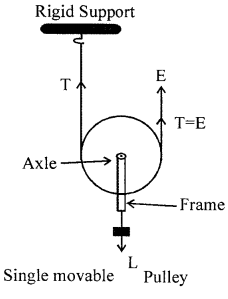Question 4.
Draw diagram of pulley system having velocity ratio (a) six (b) three
(a) Six pulleys L = 6T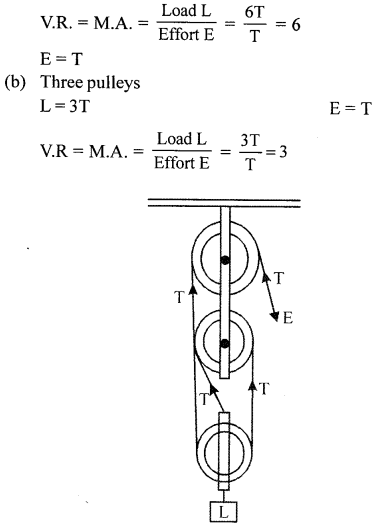Velocity Ratio (V.R.) : In a system of n pulley if the load moves up through a distance d, the effort end moves through a distance because each segment of the string supporting the load is loosened by a length d i.e. dL = d then dE = nd
∴ Velocity ratio = nd/d = n
Thus the V.R. is always equal to the number of strands of tackle (or section of the string) supporting the load.

Question 5.
How can a single pulley be used as a single movable pulley ? Explain or draw a labelled diagram.
Single movable pulley : “A pulley whose axis of rotation-is movable (not fixed in position) is a movable pulley done in Q.3.

Multiple Choice Questions

Tick (✓) the most appropriate option.

1. A single fixed pulley is used because :
(a) its efficiency is 100%
(b) it multiplies effort
(c) it multiplies speed
(d) it changes the direction of applied effort
(d) it changes the direction of applied effort

2. The actual mechanical advantage of a single movable pulley is :
(a) one
(b) two
(c) less than 2, but more than 1
(d) none of these
(c) less than 2, but more than 1

3. A sheaf pulley system has :
(a) efficiency more than 100%
(b) multiplies speed
(c) multiplies effort
(d) both (a) and (b)
(c) multiplies effort

4. A pulley system has 5 pulley in all. Its velocity ratio is :
(a) 5
(b) less than 5
(c) more than 5
(d) none of these
(a) 5

5. A single movable pulley has :
(a) velocity ratio 2, and actual mechanical advantage 2
(b) velocity ratio 2, and actual mechanical advantage less than 2
(c) velocity ratio 2, and actual mechanical advantage more than 2
(d) none of the above
(b) velocity ratio 2, and actual mechanical advantage less than 2

6. An actual pulley system always has mechanical advantage less than velocity ratio. It is because :
(a) a part of effort is wasted in overcoming friction.
(b) a part of effort is wasted in overcoming load of movable block
(c) both (a) and (b)
(d) none of these
(c) both (a) and (b)

Numerical Problems on Pulleys

Practice Problems 1

Question 1.
A pulley system has five pulley in all and is 90% efficient. Calculate

(b) the effort required to lift a load of1000 N
(c) the resistance due to the movable parts of the machine and friction.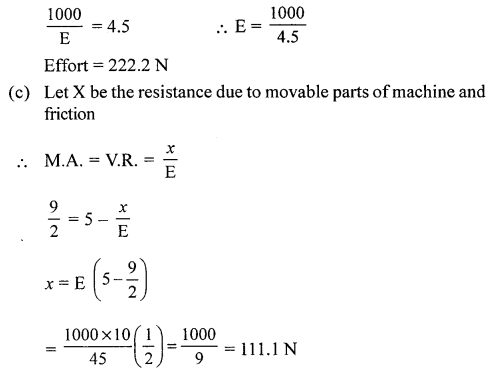Question 2.
Diagram shows a single movable pulley system.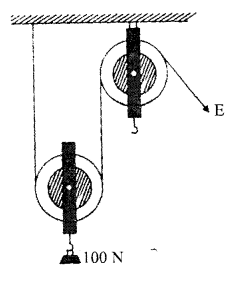(a) Mark the direction of force due to tension.
(b) What is the purpose of the fixed pulley ?
(c) If T is the tension in newtons, what is the relation between T and E ?
(d) Calculate VR of the system.
(e) Assuming efficiency is 100%, what is the mechanical advantage ?
(f) Calculate the effort E.

(a) Force due to tension is shown.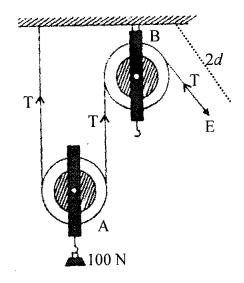(b) Purpose of fixed pulley:
(i) If effort is applied only in upward direction it is inconvenient. With the single fixed pulley B to change the direction of force is shown.
(ii) More over one can use his own weight also at effort as it will be quite convenient.
(c) T = E
(d) V.R = distance moved by effort / distance moved by load
V.R. = 2d/d = 2
∵ When free end of string is pulled up by the effort through 2d, then load is raised up through a distance d.
(e) M.A. / V.R. = η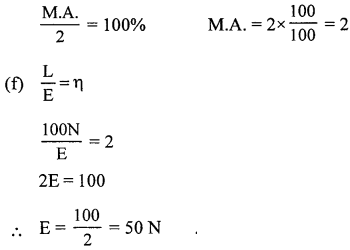Practice Problems 2

Question 1.
Diagram alongside show a pulley system when a load of 30 kgf is attached to a movable block.(a) What is the velocity ratio of pulley system ?
(b) What is the mechanical adyantage of pulley system assuming it is ideal ?
(c) What is the magnitude of effort applied ?
(d) If the pulley system is not ideal and is 60% efficient, what is the effort required ?

L = 3 kgf
If free end of string is pulled up by the effort through 3d, then the load is raised up through a distance dQuestion 2.
A pulley system has five pulleys in all, 2 in the movable block and three in fixed block, such that effort is applied in the upward direction and a load of 60 kgf is attached to the movable block.

(a) What is the velocity ratio of pulley system ?
(b) Assuming pulley system an ideal one, what is the mechanical advantage ?
(c) What is the magnitude of effort applied ?
(d) If the pulley system is not ideal but is 75% efficient, what is the effort required ?Practice Problems 3

Question 1.
A pulley system with velocity ratio 4 is used to lift a load of 100 kgf through a vertical height of IS m. The effort required to do so is 40 kgf which is applied in the downward direction. Calculate :

(a) Distance through which effort is applied
(b) Work done by the effort
(c) Mechanical advantage of pulley system
(d) Efficiency of pulley system.
(e) Number of pulleys in the upper and lower block. [Take g = 10 N kg-1]Question 2.
A pulley system with velocity ratio 3 is used to lift a load of 60 kgf through a height of 20 m. The force is applied in upward direction and its magnitude is 25 kgf. Calculate :

(a) Distance through which effort is applied
(b) Work done by the effort
(c) Mechanical advantage of pulley system
(d) Efficiency of pulley system
(e) Total number of pulleys in the fixed and movable block. [Take g = 10 N kg-1]

V.R. = 3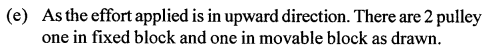Question 1.
What do you understand by the term inclined plane ?
Inclined plane : “An inclined planet is a sloping surface (like ramps or bridge over the railway tracks at a railway station) that behaves like a simple machine whose M.A. is always less than 1 ”?

Question 2.
Explain how does an inclined plane acts as a machine.
Inclined plane acts as a machine since less effort is needed in lifting a load to a higher level by moving over an inclined plane as compared to that in lifting the load directly.

Question 3.
Inclined plane acts as a machine in daily life. Give four examples.
Four examples of inclined plane acting as machine :

1. Ramp
2. Railway Bridge
3. Stair case
4. Mountainous foot path or roads.

All these act as machine as less effort is needed in lifting the load to higher level.

Question 4.
Gradient of inclination : “The ratio between the vertical distance moved by a body and the horizontal distance travelled along the inclined plane is called grade of inclination” or gradient of inclined plane.
e.g. If a body riser 10 m vertically when it moves along the inclined plane by 200 m or sin θ 10 / 200 = 1 / 20 = then inclination grade is said to be 1 in 20.
Higher is the magnitude of gradient the more difficult is the slope to climb and vice-versa.

Question 5.
What do you understand by the following terms ?

(a) gear
(b) driven wheel
(c) driving wheel.

(a) Gear : “A gear is a wheel with teeth around its rim.” Or “Is a precise device to transfer the rotatory motion from one point to the other”.
(b) Driven wheel “A wheel which receives motion from driver wheel and is connected to the load and rotates in opposite direction to driver wheel.”
(c) Driving wheel : “A gear wheel which is closer to the source of power and effort is applied to this wheel is called driving wheel”.

Question 6.
When the rotations of a driven wheel is more than a driving wheel, does it increase speed or load capacity ?
Yes, the speed of driven wheel increases when its rotation increases.

Multiple Choice Questions

Tick (✓) the most appropriate option.

1. A hill road rises up by 0.50 m, when the horizontal distance covered along the inclined plane is 20 m. The gradient of hill road is :
(a) 40 : 1
(b) 1 : 40
(c) 1 : 4
(d) 4 : 1
(b) 1 : 40

2. Which is not an example of an inclined plane ?
(a) A ramp
(b) A crowbar
(d) A stair case
(b) A crowbar

3. The mechanical advantage of an inclined plane :
(a) increases with the increase in angle of inclination
(b) decrease with the increase in the angle of inclination
(c) is unaffected with the increase in the angle of inclination
(d) none of the above
(b) decrease with the increase in the angle of inclination

4. The mechanical advantage of an inclined plane is always :
(a) less than 1
(b) more than 1
(c) equal to 1
(d) none of these
(b) more than 1

5. A gear system is used for :
(a) gain in speed
(b) gain in torque
(c) change in the direction of rotation
(d) all the above
(d) all the above

6. The driving wheel and the driven wheel of a gear system has same number of teeth. The gear system :
(a) increases the speed
(b) increases the torque
(c) changes the direction of rotation
(d) both (b) and (c)
(c) changes the direction of rotation

7. Velocity ratio (gear ratio) of a car gaining speed is :
(a) more than 1
(b) less than 1
(c) equal to one
(d) none of these
(a) more than 1

Practice Problems 1

Question 1.
An effort of 20 kgf is applied on a barrel of weight 320 kgf in order to roll it up an inclined plane, through a vertical height of 1.2 m. Calculate the length of the inclined plane.
Effort E = 20 kgfQuestion 2.
The gradient of a hill road is 1: 80. Calculate the weight of the vehicle which is pushed up an inclined plane by an effort of 150 kgf.Question 3.
A car develops a force of 500 N and climbs up a vertical distance of 200 m. If the load due to the car is 12500 N, calculate the length of the road covered by the car.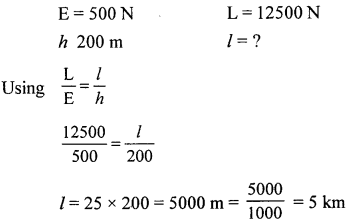Practice Problems 2

Question 1.
A car of mass 1000 kg can be lifted vertically with the help of pulley system into an open truck whose chassis is 1.2 m above the ground or can be pushed up by placing a ramp of length 4.8 m.

1. How much effort is required to lift the car directly upward?
2. How much is (a) mechanical advantage of ramp (b) effort needed to push the car up the ramp ?
3. If the actual effort needed to push the car up the ramp is 5000 N, what is the force of friction offered by the ramp?Question 2.
An oil drum of weight 75 kgf can be placed in a truck directly or by pushing it up along a wooden plank 3 m long, such that height of the floor of truck is 0.80 m above the ground level.

1. How much effort is required to life the oil drum directly upward?
2. How much is (a) mechanical advantage of inclined plane made by the wooden plank (b) effort needed to push up oil drum ?
3. If the actual effort needed to push up the oil drum along inclined is 350 N, what is the force of friction offered by the wooden plank ?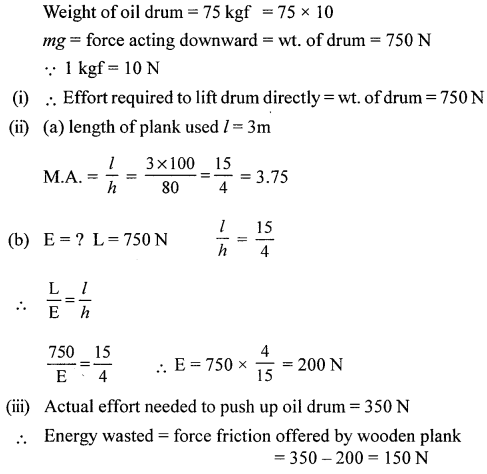Practice Problems 3

Question 1.
In a gear system, the number of teeth in the driven wheel is 16 and in the driving wheel is 48. Calculate the number of rotations made by the driven wheel for 20 rotations of driving wheel.Question 2.
The radii of the driving wheel and the driven wheel are 7 cm and 0.35 cm respectively. If the driving wheel makes 80 rotations per minute, calculate the rate of rotation of the driven wheel.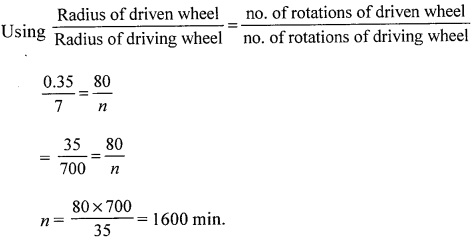Practice Problems 4

Question 1.
A gear system has one wheel with 96 teeth and other wheel with a teeth. Find (a) gain in speed (b) gain in torque which can be obtained from this system. Also find the gear ratio in each case.

(a) For gain in speed : the wheel with 96 teeth should be used as driving wheel and the wheel with 4 teeth should be used as driven wheel(b) For gain in torque : Well with 4 teeth should be used as teeth should be used as driven wheelQuestion 2.
A gear system has one wheel with 60 teeth and other wheel with 10 teeth. Calculate (a) gain in speed (b) gain in torque which can be obtained from this system. Also find the gear ratio in each case.
(a) For gain in speed : The wheel with 60 teeth should be used at driving wheel and wheel with 10 teeth as driven wheel.(b) For gain torque : The wheel with 10 teeth should be used as driving wheel : gain in torque = 60/10 = 6
Gear ratio = 10 : 60 = 1 : 6 9s less than 1

Question 3.
Define an Inclined plane.

1. It states that energy can neither be created or destroyed. It can simply change form from one form into another such that the total energy of system remains constant.
2. Electrical into sound energy,Chemical into electrical Energy.

Questions From ICSE Examination Papers

2001

Question 1.
You are required to design a machine which is a force multiplier. If the machine is a simple lever, what kind of lever will you use ? Support your answer by a simple diagram of the lever.
Class II Lever are Force Multiplier because by applying Less Effort, A Large Load Is Lifted.
ExampleQuestion 2.
Pliers and scissors are levers of the same order.

(a) Which amongst them has a mechanical advantage less than one ?
(b) State the usefulness of the lever named by you which has a mechanical advantage less than one.

(a) Class I lever can have M.A. less than 1 i.e. dL > dE example scissor
(b) So that load arm (blades move longer over the cloth (or paper) when the handle (effort arm) are moved a little.

2002

Question 3.
A cook uses a ‘fire tong’ of length 35 cm to lift a piece of burning coal of mass 200 g. If he applies his effort at a distance of 7 cm from the fulcrum, what is the effort in SI unit ? [Take g = 10 m s-1]2003

Question 4.
(a) What is the relationship between mechanical advantage and the velocity ratio for

1. An ideal machine
2. A practical machine ?

(i) Velocity Ratio = Mechanical advantage (MA)
For an Ideal machine, there is no loss of energy in any manner.
(ii) Velocity Ratio > Mechanical advantage (MA) as efficiency is less than one.
For a practical machine, output work is always less than input work as due to some loss of input energy against friction.

(b) Give two reasons why the efficiency of a single movable pulley system is less than 100%.

1. Its parts are less than frictionless.
2. Its string is not perfectly elastic.
3. Weight of movable pulley.

2004

Question 5.
(a) Scissors and shears are levers of the first order. However, the cutting edge of scissors used for cutting cloth is much longer than the cutting edge of shears used for cutting metals. Explain the difference.
To increase the load arm the blades of scissor are longer so that blades move over longer when the effort arm is moved a little.
The effort arms of shears made longer as compared to blades so that it becomes force multiplier and helps in cutting the metal sheet.
(b) A person draws water from a well using a single fixed pulley. The mass of the bucket together with water is 30 kg. If the force applied by the person is 35-0 N, calculate the mechanical advantage of the pulley. [Take g = 10 ms-2]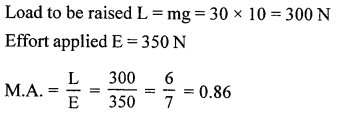2005

Question 6.
Draw a labelled sketch of a class II lever. Give one example of such a lever.
An inclined plane is a simple machine which acts as a force multiplier. It is based on the principle that the effective value of weight of a body is much less along an inclined plane than along the horizontal. It is used to carry heavy load with a small effort.Question 7.
The figure shows the combination of a movable pulley P1 with a fixed pulley P2 used for lifting up a load W.

(a) State the function of the fixed pulley P2
(b) If the free end of the string moves through a distance x, find the distance by which the load W is raised.
(c) Calculate the force to be applied at C to just raise the load W = 20 kgf, neglecting the weight of the pulley P1 and friction.(a) The fixed pulley P2 only changes the direction of the force i.e. force can be applied downwards which is more convenient.
(b) The load will be raised by a distance x/2.
(c) Distance moved by load dL = x/2
Distance moved by the effort dE = x
Effort = ?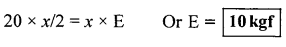2006

Question 8.
A uniform metre scale is kept in equilibrium when supported at the 60 cm mark and a mass M is suspended from the 90 cm mark. State with reason whether the weight of the scale is greater than, less than or equal to the weight of mass M.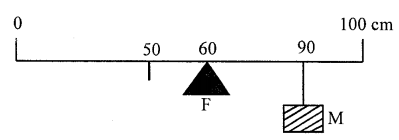Since the meter scale is uniform
∴ Its mass per unit length is same and its wt. W acts at 50 cm mark.
∴ W × (60 – 50) = M (90 – 60)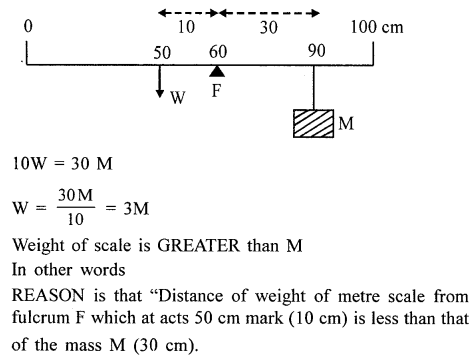Question 9.
Name the type of single pulley that can act as a force multiplier. Draw a labelled diagram of the above named pulleyLever of class second acts as force multiplier.

Question 10.
A pulley system has a velocity ratio of 4 and an efficiency 90%.”
Calculate :

(a) Mechanical advantage of the system.
(b) Effort required to raise a load of 300 N by the system.2007

Question 11.
Which class of levers has a mechanical advantage always greater than one ? What change can be brought about in this lever to increase its mechanical advantage ?
Class II levers is always greater than 1 To increase M.A. of the lever, effort arm should be longer than

Question 12.
Write an expression to show the relationship between mechanical advantage, velocity ratio and efficiency for a simple machine.
Expression of relation M.A, V.R and efficiency is M.A.
Efficiency η = M.A / V.R.

Question 13.
A block and tackle pulley system has a velocity ratio 3.

(a) Draw a labelled diagram of this system. In your diagram, indicate clearly the points of application and the directions of the load and effort.
(b) Why should the lower block of this pulley system be of negligible weight ?

(a) Block and Tackle Pulley System has VR = 3 (odd)
∴ Number of pulleys = 3
2 in block and 1 in tackle.
The labelled diagram is as shown in the fig.MA = Total number of pulleys in the two blocks i.e., 3
(b) The weight of lower block should be negligible so that efficiency should be more.

2008

Question 14.
Copy the diagram of the forearm given below, indicate the positions of Load, Effort and Fulcrum.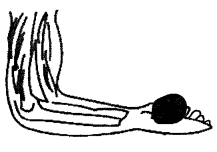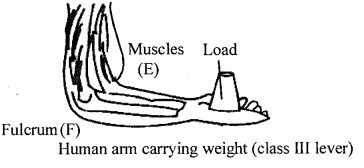Question 15.
(a) Draw a labelled diagram of a block and tackle system of pulleys with two pulleys in each block. Indicate the directions Of the load, effort and tension in the string.

(b)
Write down the relation between the load and the effort of the pulley system.
(a) A pulley system with two pulleys in each block.2009

Question 16.
(a) With reference to the terms mechanical advantage, velocity ratio and efficiency of a machine, name the term that will not change for a machine of a given design.
(b) Define the term stated by you in part (i).
(a) The term is velocity ratio as M.A depends on load to be raise and effort applied keep on changing and Efficiency also changes due to friction between pulley and string and also pulley and axle.
(b) V.R. : “The ratio of velocity of effort to the velocity of load.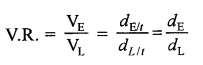V.R. is “the ratio of displacement of effort to displacement of laod.”

Question 17.
A pulley system comprises of two pulleys, one fixed and the other movable.
(a) Draw a labelled diagram of the arrangement and show clearly the directions of all the forces acting on it.(b) What change can be made in the movable pulley of this system to increase the mechanical advantage of the system ?
To increase the mechanical advantage of the system : Weight of the pulley and the string should be made negligible (as less as possible)

2010

Question 18.

(a) Why is the mechanical advantage of a lever of the second order always greater the one ?
(b) Name the type of single pulley that has a mechanical advantage greater than one.

(a) The mechanical advantage of second order levers is always more than 1 because the effort arm is always longer than the load arm.
(b) A single movable pulley has MA greater than 1Question 19.

(a) Write a relation expressing the mechanical advantage of a lever.
(b) Give two reasons as to why the efficiency of a single movable pulley system is always less than 100%.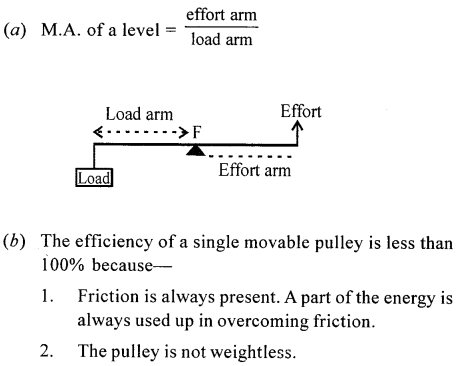2011

Question 20.
(a) A man can open a nut by applying a force of 150 N by using a lever handle Of length 0.4 m. What should be the length of the handle if he is able to open it by applying a force of 60 N ?
(b) Name a machine which can be used to

1. multiply force
2. change the direction of force applied

(c) Diagram shows a lever in use.1. To which class of lever does it belong ?
2. If FA = 40 cm, AB = 60 cm, then find the mechanical advantage of the lever.Question 21.
(a) What is meant by an ideal machine ?
(b) Write a relationship between the mechanical advantage (M.A.) and velocity ratio (V.R.) of an ideal machine.
(c) A coolie carrying a load on his head and moving on a frictionless horizontal platform does no work. Explain the reason why.
(a) In an ideal machine, the work output is equal to the
work input i.e., the efficiency of an ideal machine is 100%. There is no loss of energy in any manner in Ideal Machine.2012

Question 22.
State the class of levers and the relative positions of load (L), effort (E) and fulcrum (F) in each of the following cases.

(a) A bottle opener
(b) Sugar tongs.

(a) In case of bottle opener the load (L) acts in the middle of metal crown, the fulcrum (F) at the edge of metal crown and effort (E) at the end of the handle. It is a lever of second order.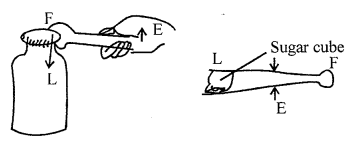(b) Sugar tongs is a lever of third order. The sugar cube at the ends of tongs acts as load (L), the effort (E) acts in the middle and fulcrum (F) acts at its end.

2013

Question 23.
(a) A type of single pulley is very often used as a machine even though it does not give any gain in mechanical advantage.

1. Name the type of pulley used
2. For what purpose is such a pulley used ?

1. Single fixed pulley.
2. It helps in changing the direction of applying efforts.

(b) (i) In what way does an ‘Ideal machine’ differ from a ‘Practical machine’ ?
(ii) Can a simple machine act as a force multiplier and a speed multiplier at the same time?
(i) An ideal machine is the one whose parts are frictionless as well as weightless, such that its mechanical advantage is equal to its velocity ratio.
A practical machine is the one whose parts are neither frictionless nor weightless. Furthermore, its mechanical advantage is always less than its velocity ratio.
(ii) No, it can be either speed multiplier or force multiplier.

(c) Which class of lever found in the human body is being used by a boy

1. when he holds a load on the palm of his hand
2. when he raises the weight of his body on his toes ?

1. Lever of third order.
2. Lever of second order

Question 24.
A block and tackle system of pulley’s a velocity ratio 4.

(a) Draw a labelled diagram of the system indicating clearly the points of application and directions of a load and effort.
(b) What is the value of the mechanical advantage of the given pulley system if it is an ideal pulley system ?

(a)(b) Mechanical advantage will be equal to the number of pulleys engaged in a given pulley system.

2014

Question 25.
(a) What is the principle of an ideal machine?
An ideal machine is that in which there is no dissipation of energy in any manner. The work output is equal to the work input.
Principle of Ideal Machine :
Output energy = Input energy

(b)
Draw a diagram to show how a single pulley can be used so as to have its ideal M.A. = 2.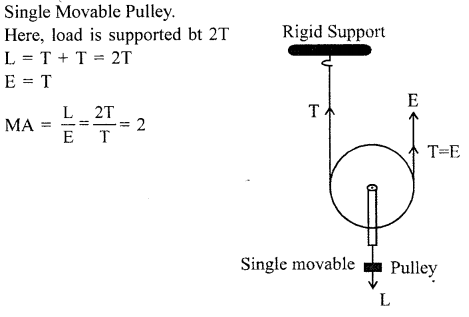(c) Derive a relationship between mechanical advantages, velocity ratio and efficiency of a machine.2015

Question 26.
(a) Draw a simplified diagram of a lemon crusher, indicting direction of load and effort.
The diagram below shows a lemon crusher indicating the direction of effort (E) and load (L).(b) A block and tackle system has V.R. = 5.

1. Draw a neat labelled diagram of a system indicating the direction of its load and effort.
2. Rohan exerts a pull of 150 kgf. What is the maximum load he can raise with this pulley system if its efficiency = 75%?2016

Question 27.
(a) With reference to the terms Mechanical Advantage, Ve locity Ratio and efficiency of a machine, name and define the term that will not change for machine of a given design
(b) From the diagram given below, answer the question that follow :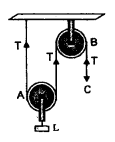• What kind of pulleys are A and B ?
• State the purpose of pulley B.
• What effort has to be applied at C just raise the load L = 20 kgf ? (Neglect the weight of pulley A and friction)

(c) A pulley system has three pulleys. A load of 120 N is overcome by applying an effort of 50 N. Calculate the Mechanical Advantage and Efficiency of this system.